# Manual 4 Jee Linalg 2d

### Manual%204%20jee_linalg_2d

User Manual:

Open the PDF directly: View PDF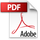.
Page Count: 4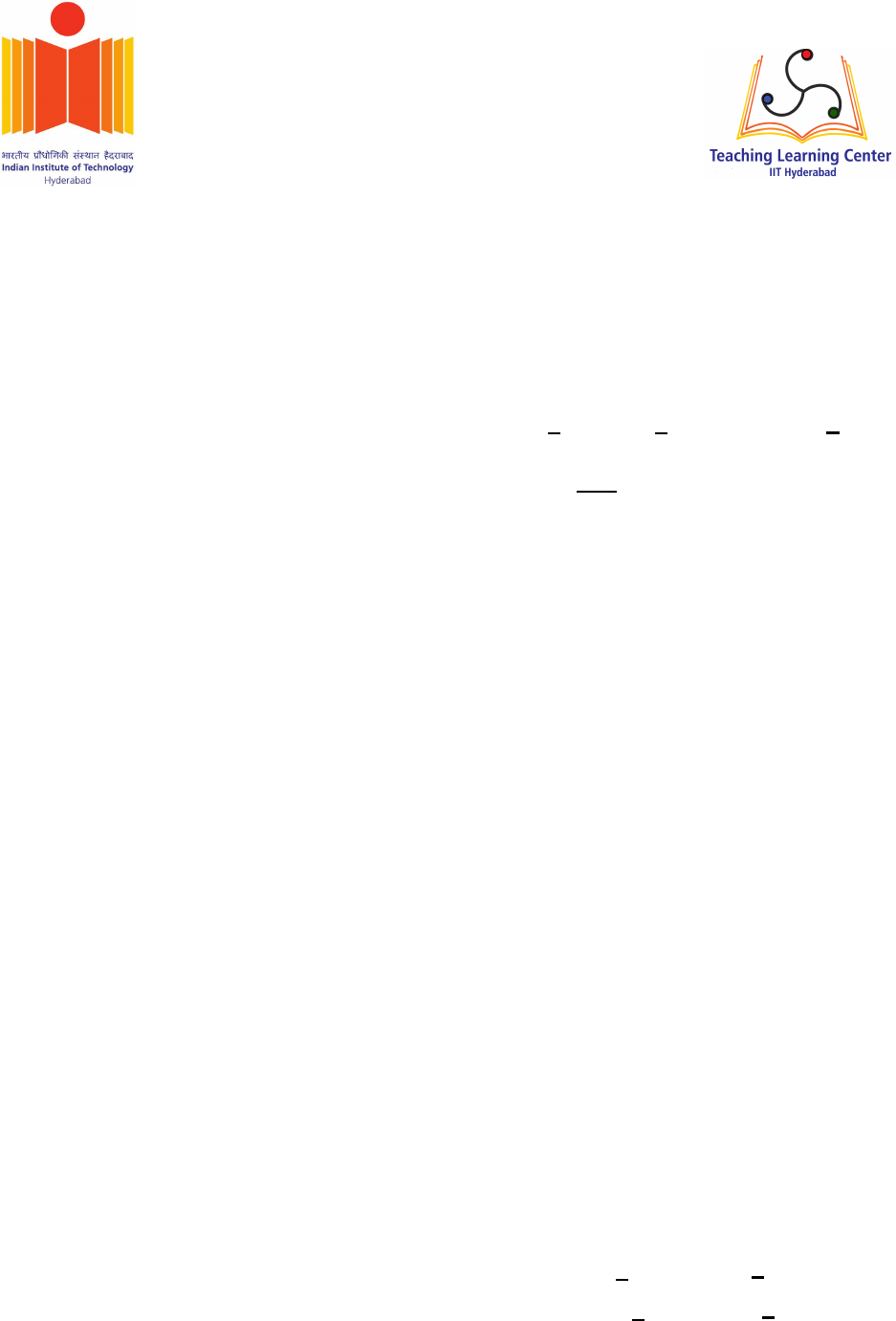1
JEE Problems in Linear
Algebra: 2D
Abstract—A collection of problems from JEE mains
papers related to 2D coordinate geometry are available
in this document. These problems should be solved using
linear algebra.
1. Tangent and normal are drawn at
P= 16
16!(1)
on the parabola
xT 0 0
0 1!x+16 0x=0 (2)
which intersect the axis of the parabola at
Aand Brespectively. If Cis the centre of
the circle through the ponts P A and B, ﬁnd
tan CPB.
2. A circle passes through the points 2
3!and 4
5!.
If its centre lies on the line
1 4x+3=0 (3)
3. Two parabolas with a common vertex and
with axes along x-axis and y-axis, respectively,
intersect each other in the ﬁrst quadrant. If the
length of the latus rectum of each parabola is
3, ﬁnd the equation of the common tangent to
the two parabolas.
4. If the tangents drawn to the hyperbola
xTVx+1=0 (4)
where
V= 1 0
04!(5)
intersect the coordinate axes at the distinct
points Aand B, ﬁnd the locus of the mid point
of AB.
5. βis one of the angles between the normals to
the ellipse
xTVx=9 (6)
where
V= 1 0
0 3!(7)
at the points
3 cos θ
3 sin θ!, 3 sin θ
3 cos θ!, θ 0,π
2,(8)
then ﬁnd 2 cot β
sin 2θ.
6. The sides of a rhombus ABC are parallel to the
lines
11x+2=0 (9)
71x+3=0.(10)
If the diagonals of the rhombus intersect at
P= 1
2!(11)
and the vertex A(dierent) from the origin is
on the y-axis, then ﬁnd the ordinate of A.
7. Tangents drawn from the point 8
0!to the
parabola
xT 0 0
0 1!x+8 0x=0 (12)
touch the parabola at Pand Q. If Fis the focus
of the parabola, then ﬁnd the area of PFQ.
8. A normal to the hyperbola
xT 4 0
09!x=36 (13)
meets the coordinate axes xand yat Aand
Brespectively. If the parallelogram OABP is
formed, ﬁnd the locus of P.
9. Find the locus of the point of intersection of
the lines
21x+42k=0 (14)
2k kx42=0 (15)
10. If a circle C,whose radius is 3, touches exter-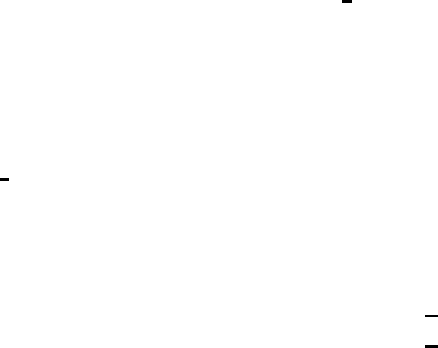2
nally the circle
xTx+24x=4 (16)
at the point 2
2!, then ﬁnd the length of the
intercept cut by this circle Con the x-axis.
11. Let Pbe the parabola
xT 1 0
0 0!x+0 4x=0 (17)
Given that the distance of Pfrom the centre of
the circle
xTx+ 6
0!x+8=0 (18)
is minimum. Find the equation of the tangent
to the parabola at P.
12. The length of the latus rectum of an ellipse
is 4 ad the distance between a focus and its
nearest vertex on the major axis is 3
2. Find its
eccentricity.
13. A square, of each side 2, lies above the x-axis
and has one vertex at the origin. If one of the
sides passing through the origin makes an angle
30with the positive direction of the x-axis,
then ﬁnd the sum of the x-coordinates of the
vertices of the square.
14. A line drawn through the point
P= 4
7!(19)
cuts the circle
xTx=9 (20)
at the points Aand B. Find PA.PB.
15. Find the eccentricity of an ellipse having centre
at the origin, axes along the coordinate axes
and passing through the points
P= 4
1!,Q= 2
2!.(21)
16. m1x+c=0 is the normal at a point on
the parabola
xT 0 0
0 1!x8 0x=0=0 (22)
whose focal distance is 8. Find |c|.
17. Find the locus of the point of intersection of
the straight lines
t2x3t=0 (23)
12tx+3=0 (24)
18. The common tangents to the parabola
xT 1 0
0 0!x 0
4!x=0=0 (25)
intersect at the point P. Find the distance of P
from the origin.
19. Consider an ellipse, whose centre is at the
origin and its major axis is along the x-axis. If
its eccentricity is 3
5and the distance between its
foci is 6, then ﬁnd the area of the quadrilateral
inscribed in the ellipse, with the vertices as the
vertices of the ellipse.
20. Let kbe an integer such that the triangle with
vertices k
3k!, 5
k!, k
2!(26)
has area 28. Find the orthocentre of this trian-
gle.
21. A hyperbola passes through the point
P= 2
3!(27)
and has foci at ±2
0!. Find the equation of the
tangent to this hyperbola at P.
22. If an equlateral triangle, having centroid at the
origin, has a side along the line
1 1x=2,(28)
then ﬁnd the area of this triangle.
23. Find the equation of the circle, which is the
mirror image of the circle
xTx2 0x=0 (29)
in the line 1 1x=3.(30)
24. Find the product of the perpendiculars drawn
from the foci of the ellipse
xT 25 0
0 9!x=225 (31)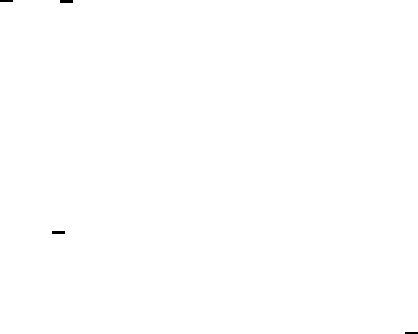3
upon the tangent to it at the point
1
2 3
53!(32)
25. Find the equation of the normal to the hyper-
bola
xT 9 0
016!x=144 (33)
drawn at the point
8
33!(34)
26. Two sides of a rhombus are along the lines
11x+1=0 (35)
71x5=0.(36)
If its diagonals intersect at
1
2!,(37)
ﬁnd its vertices.
27. Find the locus of the centres of those circles
which touch the circle
xTx81 1x=4 (38)
and also touch the x-axis.
28. One of the diameters of the circle, given by
xTx+22 3x=12 =0 (39)
is a chord of a circle S, whose centre is at
3
2!.(40)
29. Let Pbe the point on the parabola
xT 0 0
0 1!x8 0x=0 (41)
which is at a minimum distance from the centre
Cof the circle
xTx+0 12x=1 (42)
Find the equation of the circle passing through
Cand having its centre at P.
30. Find the eccentricity of the hyperbola whose
length of the latus rectum is equal to 8 and the
length of its conjugate axis is equal to half the
distance between its foci.
31. A variable line drawn through the intersection
of the lines
4 3x=12 (43)
3 4x=12 (44)
meets the coordinate axes at Aand B, then ﬁnd
the locus of the midpoint of AB.
32. The point 2
1!(45)
is translated parallel to the line
11x=4 (46)
by 2 3 units. If the new point Qlies in the
third quadrant, then ﬁnd the equation of the
line passing through Qand perpendicular to L.
33. A circle passes through
2
4!(47)
and touches the y-axis at
0
2!.(48)
Which one of the following equations can
represent a diameter of this circle?
a) 4 5x=6
b) 23x+10 =0
c) 3 4x=3
d) 5 2x+4=0
34. Let aand brespectively be the semi-transverse
and semi-conjugate axes of a hyperbola whose
eccentricity satisﬁes the equation
9e218e+5=0 (49)
If
S= 5
0!(50)
is a focus and
5 0x=9 (51)
is the corresponding directrix of this hyperbola,
then ﬁnd a2b2.
35. A straight line through the origin Omeets the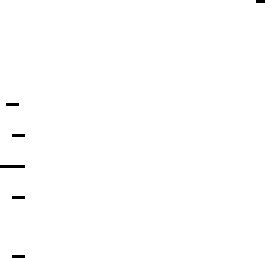4
lines
4 3x=10 (52)
8 6x+5=0 (53)
at Aand Brespectively. Find the ratio in which
Odivides AB.
36. Find the equation of the tangent to the circle,
at the point 1
1!,(54)
whose centre is the point of intersection of the
straight lines
2 1x=3 (55)
11x=1 (56)
37. Pand Qare two distinct points on the parabola
xT 0 0
0 1!x4 0x=0 (57)
with parameters tand t1respectively. If the
normal at Ppasses through Q, then ﬁnd the
minimum value of t2
1.
38. A hyperbola whose transverse axis is along the
major axis of the conic
xTVx=51 (58)
where
V= 3 0
0 27!(59)
and has vertices at the foci of this conic. If the
eccentricity of the hyperbola is 3
2, which of the
following points doesnot lie on it?
a) 0
2!
b) 5
22!
c) 10
23!
d) 5
23!
39. A tangent at a point on the ellipse
xTVx=51 (60)
where
V= 3 0
0 27!(61)
meets the coordinate axes at Aand B. If Obe
the origin, ﬁnd the minimum area of OAB.